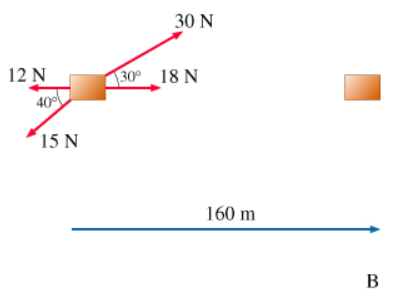# Problem: Find the work W done by the 18-newton force.

###### FREE Expert Solution

W = Fd cosθ

Here, d = 160 m, F = 18 N—parallel to d, so θ=180°

84% (429 ratings)###### Problem Details

Find the work W done by the 18-newton force.